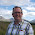## Tuesday, 25 November 2014

### Excel Tip: The PMT function - calculating loan payments in Excel

Excel has a baffling array of functions under the heading of "Financial", many of which are only likely to be used by financial analysts. However, over the next few weeks, I will tell you about a few that can be very useful to the rest of us.

This week, I want to introduce you to the PMT function, which allows you to calculate payments on a loan.

Before we get into how the PMT function works, it is useful to note what types of loan it will work for (and what types it won't!).

The function works for loans with a constant interest rate and regular payments of the same amount. The only exception to this is that it does allow for a 'balloon payment' at the end of the loan.

If this is the nature of the loan payment you want to calculate, then the PMT function will work for you.

Essentially, you feed the function the loan details, and it returns the regular payment amount.

The syntax of the PMT function is:

=PMT(Interest Rate,No of Periods,Present Value,[Future Value],[Type])

The last two arguments are optional and we'll come to those later in this post.

The three mandatory arguments are explained below:

Interest Rate: This is the interest rate per payment period, so if you have an annual interest rate of 6% with monthly payments, the Interest Rate entered shound be 0.5% (i.e. 6% divided by 12).

No of Periods: This is the number of periods or payments of the loan. A 5 year loan with monthly payments would be 60, for example.

Present Value: This is the value, today, of the loan and for a new loan will be the loan advance amount.

So, say we have a £10,000 loan over 5 years with monthly payments and an annual interest rate of 12%:

Interest Rate = 12%/12 = 1% or .01
No of Periods = 5 x 12 = 60
Present Value = £10,000

So our function becomes:

=PMT(.01,60,10000)

and returns a monthly payment of £222.44. Note that the function actually returns -222.44 as we entered Present Value as  positive figure. If the receipt of £10,000 is positive, then for consistency, the payment returned is negative. We could enter the £10,000 as a negative number (i.e. a negative payment), which would return a positive payment.

In reality, we wouldn't normally enter these figures into the function directly, more likely we would hold them in cells so that we could experiment with changing their values. and the function would be more like =PMT(B1,B2,B3), where Interest Rate, No of Periods and Present Value were held in cells B1, B2 and B3 respectively.

The final two (optional) arguments work as follows:

[Future Value]: This is where you would enter a 'balloon' payment, or any balance that you wish to remain outstanding at the end of the period you are looking at. You might have a final 'balloon' payment of £5,000, and therefore the payment calculated would need to leave exactly £5,000 outstanding when No of Periods comes to an end. Alternatively, you might recalculate payments annually to hit a specific target balance at the end of each year. This should be entered with the same sign as the payments (i.e. the opposite sign to Present Value). This arguments is treated as zero if omitted, i.e. the loan will be completely paid off after all payments are made.

[Type]: This is a 1 or a 0 and indicates when in each period the payments are made. If this is 0, or omitted, the calculation assumes that payments are made at the end of each of the periods in No of Periods. Enter 1 for this argument if payments are made at the start of each period.

If you enjoyed this post, go to the top of the blog, where you can subscribe for regular updates and get two freebies "The 5 Excel features that you NEED to know" and "30 Chants for Better Charts".

1.2.1.